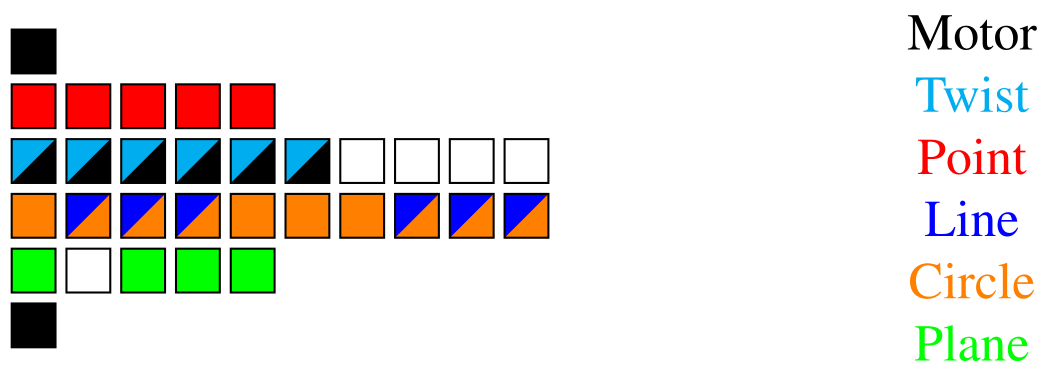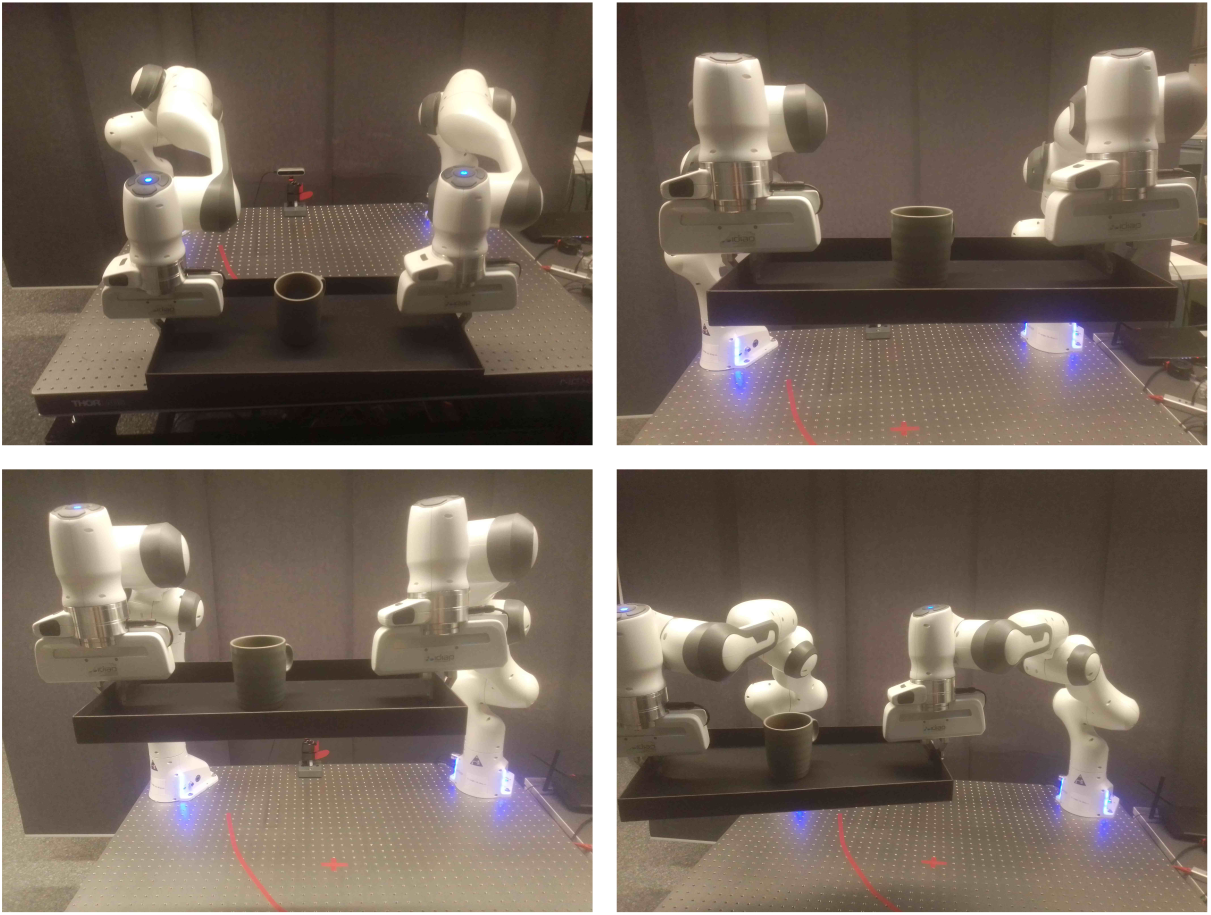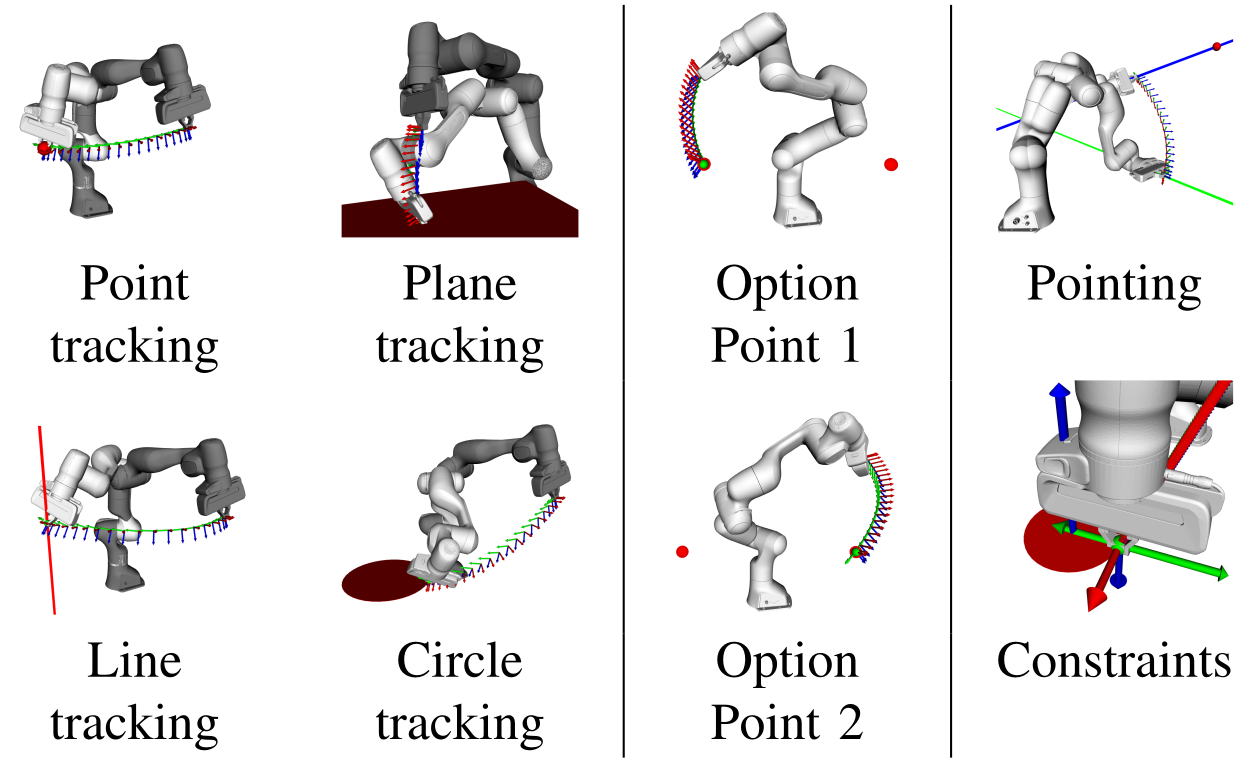# GEOMETRIC ALGEBRA

## INTRODUCTION

For a long time robots were confined to industrial tasks that are well defined and very static. Currently we have a large push to move robots to human environments, which means there is the need for interaction either with humans or with other robots. This can be as kitchen aids, in elderly care or to drive us around. These environments can be very dynamic or even chaotic. So there is a lot of added complexity. To tackle that we introduce different abstraction layers that allow a more precise reasoning on a semantic level. The issue with that are different mathemetical representations that reintroduce complexity or unreliability when we need to convert between representations. Many problems in robotics are fundamentally problems of geometry, so choosing a represention with a strong geometrical and physical meaning. Geometric algebra is such a a unified mathematical representation.

## PUBLICATIONSGeometry is a fundamental part of robotics and there have been various frameworks of representation over the years. Recently, geometric algebra has gained attention for its property of unifying many of those previous ideas into one algebra. While there are already efficient open-source implementations of geometric algebra available, none of them is targeted at robotics applications. We want to address this shortcoming with our library gafro. This article presents an overview of the implementation details as well as a tutorial of gafro, an efficient c++ library targeting robotics applications using geometric algebra. The library focuses on using conformal geometric algebra. Hence, various geometric primitives are available for computation as well as rigid body transformations. The modeling of robotic systems is also an important aspect of the library. It implements various algorithms for calculating the kinematics and dynamics of such systems as well as objectives for optimisation problems. The software stack is completed by python bindings in pygafro and a ROS interface in gafro_ros.In this work, we are presenting an extension of the cooperative dual-task space (CDTS) in conformal geometric algebra. The CDTS was first defined using dual quaternion algebra and is a well established framework for the simplified definition of tasks using two manipulators. By integrating conformal geometric algebra, we aim to further enhance the geometric expressiveness and thus simplify the modeling of various tasks. We show this formulation by first presenting the CDTS and then its extension that is based around a cooperative pointpair. This extension keeps all the benefits of the original formulation that is based on dual quaternions, but adds more tools for geometric modeling of the dual-arm tasks. We also present how this CGA-CDTS can be seamlessly integrated with an optimal control framework in geometric algebra that was derived in previous work. In the experiments, we demonstrate how to model different objectives and constraints using the CGA-CDTS. Using a setup of two Franka Emika robots we then show the effectiveness of our approach using model predictive control in real world experiments.Many problems in robotics are fundamentally problems of geometry, which lead to an increased research effort in geometric methods for robotics in recent years. The results were algorithms using the various frameworks of screw theory, Lie algebra and dual quaternions. A unification and generalization of these popular formalisms can be found in geometric algebra. The aim of this paper is to showcase the capabilities of geometric algebra when applied to robot manipulation tasks. In particular the modelling of cost functions for optimal control can be done uniformly across different geometric primitives leading to a low symbolic complexity of the resulting expressions and a geometric intuitiveness. We demonstrate the usefulness, simplicity and computational efficiency of geometric algebra in several experiments using a Franka Emika robot. The presented algorithms were implemented in c++20 and resulted in the publicly available library gafro. The benchmark shows faster computation of the kinematics than state-of-the-art robotics libraries.